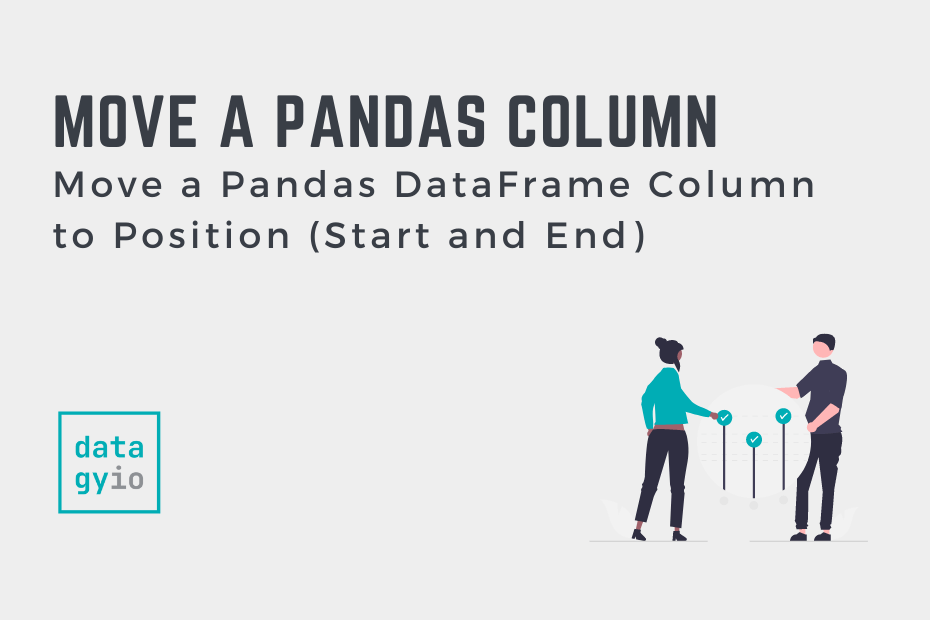# Pandas## Move a Pandas DataFrame Column to Position (Start and End)

Being able to work with and manipulate Pandas DataFrames is an essential skill for anyone working in data analysis or data science. As Pandas becomes the ubiquitous tool for data analysts, being able to get DataFrames ready for presentation is… Read More »Move a Pandas DataFrame Column to Position (Start and End)## Pandas: Replace NaN with Zeroes

Working with missing data is an essential skill for any data analyst or data scientist! In many cases, you’ll want to replace your missing data, or NaN values, with zeroes. In this tutorial, you’ll learn how to use Pandas to… Read More »Pandas: Replace NaN with Zeroes## Pandas Groupby and Aggregate for Multiple Columns

The Pandas groupby method is incredibly powerful and even lets you group by and aggregate multiple columns. In this tutorial, you’ll learn how to use the Pandas groupby method to aggregate multiple columns. The syntax of the method can be… Read More »Pandas Groupby and Aggregate for Multiple Columns## How to Convert JSON to CSV in Python

JavaScript Object Notation (JSON) is one of the most common formats you’ll encounter when working with data – especially web data. Being able to convert the format into other formats, such as CSV, is an important skill. In this tutorial,… Read More »How to Convert JSON to CSV in Python## Convert a Pandas DataFrame to a Pickle File

Pickle files are serialized data structures that allow you to maintain data state across sessions. Pickle files are incredibly common in data science. In this tutorial, you’ll learn how to serialize a Pandas DataFrame to a Pickle file. This is… Read More »Convert a Pandas DataFrame to a Pickle File

## Convert a Pandas DataFrame to a NumPy Array

Pandas provides simplicity and flexibility in converting a Pandas DataFrame to a NumPy array. Being able to convert between the Pandas format into a NumPy format is a versatile skill for any data analyst or data scientist. The Pandas .to_numpy()… Read More »Convert a Pandas DataFrame to a NumPy Array## Convert a Pandas DataFrame to JSON

In this tutorial, you’ll learn how to convert a Pandas DataFrame to a JSON object and file using Python. Most programming languages can read, parse, and work with JSON. Because of this, knowing how to convert a Pandas DataFrame to… Read More »Convert a Pandas DataFrame to JSON## Convert a Pandas DataFrame to a Dictionary

In this post, you’ll learn how to use Python to convert a Pandas DataFrame into a dictionary. Because Pandas DataFrames are complex data structures, there are many different ways in which this can be done. This post explores all of… Read More »Convert a Pandas DataFrame to a Dictionary## Convert a Pandas DataFrame to a List

In this post, you’ll learn how to convert a Pandas DataFrame to a list, including a list of lists, a list of tuples, and a list of dictionaries. Being able to convert a Pandas DataFrame to different formats allows you… Read More »Convert a Pandas DataFrame to a List## NumPy argmin(): Get Index of the Min Value in Arrays

In this tutorial, you’ll learn how to master the NumPy argmin() function to find the index position of the minimum value in a NumPy array. The np.argmin() function provides incredible functionality for working with one-dimensional and multi-dimensional arrays. You’ll also… Read More »NumPy argmin(): Get Index of the Min Value in Arrays